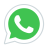# Laplace Transform

Ordinary and partial differential equations describe the way certain quantities vary with time, such as the current in an electrical circuit, the oscillations of a vibrating membrane, or the flow of h...
created by zeeshan saleem
Last updated 2021-12-26
Language: English
\$50
\$70
This course has
Access on mobile
Certificate of Completion

• Laplace Transform

## Course Requirements

1. Differential Equations

## Which Students must take this course

1. Bsc in Mathmatics Student
2. Msc in Mathmatical Student
3. BS Engineers
4. MS Engineers
5. PHD Students

## Course Content

Laplace Introduction
00:04:10
Laplace Transform in a Linear Operator
00:02:56
Laplace Transform of a Constant Function
00:11:10
Laplace Transform of a Monomial Function
00:10:56
Laplace Transform of a Exponential Function
00:04:49
Laplace Transform of a Piecewise Continuous Function (Part-1)
00:12:11
Laplace Transform of a Piecewise Continuous Function (Part-2)
00:12:11
Laplace Transform of Sinkt
00:10:46
Laplace Transform of coshkt
00:08:46
Laplace Transform of Sumtopro
00:06:15
First Translation Theorem
00:05:28
Exp of First Translation Theorem
00:07:27
Laplace Transform of Sinhktcoshkt
00:03:43
Laplace Transform of coshktSinhktexp
00:04:52
Units Function
00:06:06
Second Translation Function
00:07:51
Exp of Second Translation Function
00:08:20
Laplace Transform of Exponential function by Direct Method
00:04:32
Laplace Transform of Monomial by Direct Method
00:06:35
Laplace Transform of Sinktcoskt by Direct Method
00:04:39
Laplace Transform of Monomial by Iterative Process
00:10:12
Proof of Derivative of Transformation
00:08:26
Example of Derivative of Transformation
00:10:45

## Description

Ordinary and partial differential equations describe the way certain quantities vary with time, such as the current in an electrical circuit, the oscillations of a vibrating membrane, or the flow of heat through an insulated conductor. These equations are generally coupled with initial conditions that describe the state of the system at time t = 0. A very powerful technique for solving these problems is that of the Laplace transform, which literally transforms the original differential equation into an elementary algebraic expression. This latter can then simply be transformed once again, into the solution of the original problem. This technique is known as the “Laplace transform method.” We will study the following: Basic Definition of Laplace Transform and its Existence, Exponential Order, Sufficient conditions for Existence, Laplace Transform is a linear Operator, Laplace Transform of a Constant Function, Laplace Transform of a Monomial Function, Laplace Transform of a polynomial Function, Laplace Transform of a Exponential Function, Laplace Transform of a Sine and cosine Function, Laplace Transform of a Hyperbolic Sine and cosine Function, Laplace Transform of a composition of a Function, Laplace Transform of a Piece-wise Function, Laplace Transform by First Translation Theorem, Unit Step Function, Laplace Transform by Second Translation Theorem, Derivatives of Transforms, Convolution Theorem and also inverse of Laplace.

Message 1 sec ago
URL has been copied